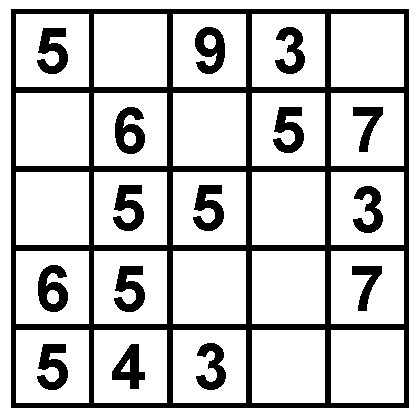## HOT KEY PUZZLE FOR JUNE 2003This is the diagram you need and can download for the June 2003 Hot Key puzzle. The puzzle is as follows:

Each of the 5 columns, 5 rows and the two main diagonals add up to the same total. Can you fill in the missing numbers?

SOLUTION

The diagonal 5555x and the vertical 4556x both sum to 20+x and x is present twice in the top row (5x93x) so 17+2x = 20+x which makes x=3. So all the rows and columns add up to 23. The rest is easy.

[home] [back]